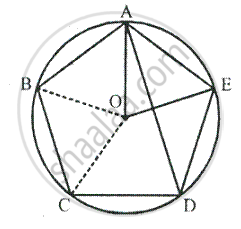Share

# In a Regular Pentagon Abcde, Inscribed in a Circle; Find Ratio Between Angle Eda and Angle Adc. - Mathematics

Course

#### Question

In a regular pentagon ABCDE, Inscribed in a circle; find ratio between angle EDA and angle ADC.

#### SolutionArc AE subtends ∠AOE at the centre and
∠ADE at the remaining part of the circle.

∴ ∠ADE  = 1/2 xx 72°
= 36                   [central angle is a regular pentagon at O]
= 36° + 36° + 72°

Is there an error in this question or solution?